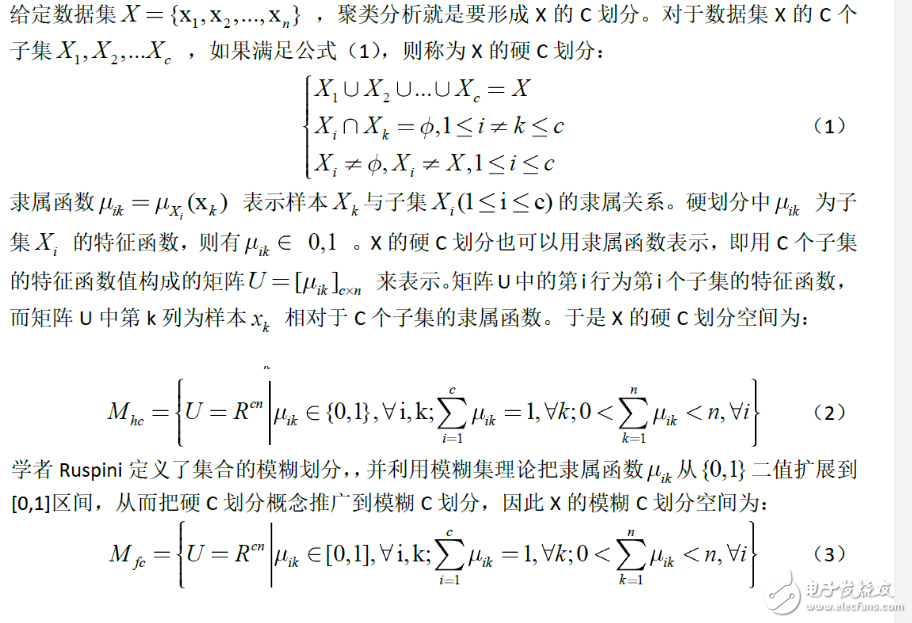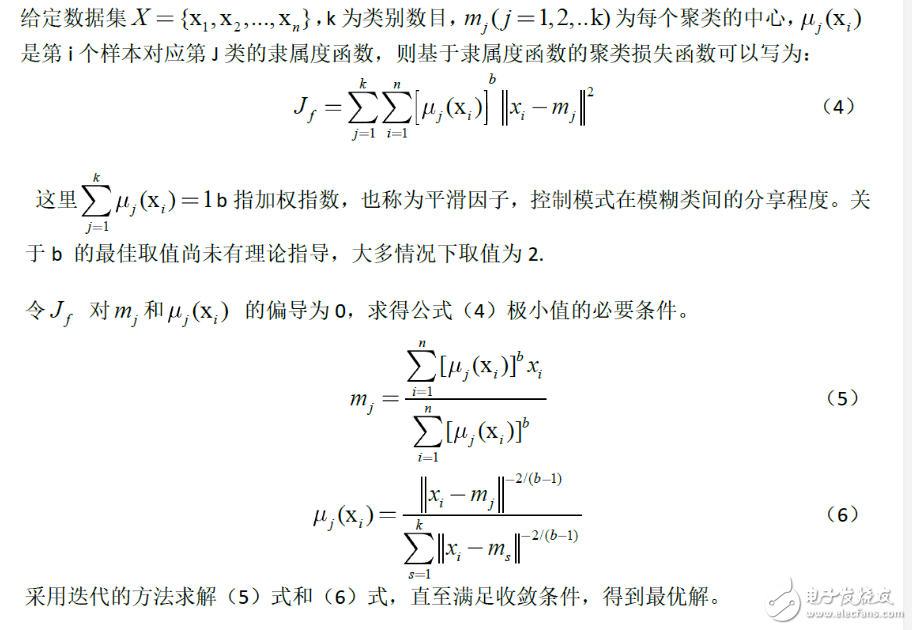• 2021-02-05 23:13:20

## C均值聚类算法FCM matlab实现

更多相关内容
• c均值聚类算法matlab代码ISO标准 ISO-SPLIT是一种有效的聚类算法，可以处理中低维度的未知数量的单峰聚类，而无需任何用户可调整的参数。 它基于对单峰性的重复测试-使用等渗回归和改进的Hartigan dip测试-应用于...
• c均值聚类算法matlab代码fcm_m 这是matlab代码中FCM聚类方法的一些变体。 您可以参考以下文章以进一步了解：“一种鲁棒的模糊局部信息C均值聚类算法”
• 聚类分割算法是图像处理中的基本操作，文件中包含了使用模糊C-均值实现聚类分割的算法原理以及MAtlab源程序 聚类分割算法是图像处理中的基本操作，文件中包含了使用模糊C-均值实现聚类分割的算法原理以及MAtlab源...
• c均值聚类算法matlab代码 iqm代表反向量化的k均值（IQ-means），并实现了一种用于快速近似聚类的方法。 执照 iqm拥有2句BSD许可证。 请参阅文件以获取完整的许可证文本。 目录结构 iqm主要由Matlab .m文件组成，但还...
• function [center, U, obj_fcn] = FCMClust(data, cluster_n, options)% FCMClust.m 采用模糊C均值对数据集data聚为cluster_n类% 用法：% 1. [center,U,obj_fcn] = FCMClust(Data,N_cluster,options);% 2. [center,U...

function [center, U, obj_fcn] = FCMClust(data, cluster_n, options)

% FCMClust.m 采用模糊C均值对数据集data聚为cluster_n类

% 用法：

% 1. [center,U,obj_fcn] = FCMClust(Data,N_cluster,options);

% 2. [center,U,obj_fcn] = FCMClust(Data,N_cluster);

% 输入：

% data ---- nxm矩阵,表示n个样本,每个样本具有m的维特征值

% N_cluster ---- 标量,表示聚合中心数目,即类别数

% options ---- 4x1矩阵，其中

% options(1): 隶属度矩阵U的指数，>1 (缺省值: 2.0)

% options(2): 最大迭代次数(缺省值: 100)

% options(3): 隶属度最小变化量,迭代终止条件(缺省值: 1e-5)

% options(4): 每次迭代是否输出信息标志(缺省值: 1)

% 输出：

% center ---- 聚类中心

% U ---- 隶属度矩阵

% obj_fcn ---- 目标函数值

% Example:

% data = rand(100,2);

% [center,U,obj_fcn] = FCMClust(data,2);

% plot(data(:,1), data(:,2),'o');

% hold on;

% maxU = max(U);

% index1 = find(U(1,:) == maxU);

% index2 = find(U(2,:) == maxU);

% line(data(index1,1),data(index1,2),'marker','*','color','g');

% line(data(index2,1),data(index2,2),'marker','*','color','r');

% plot([center([1 2],1)],[center([1 2],2)],'*','color','k')

% hold off; %%%%%%%%%%%%%%%%%%%%%%%%%%%%%%%%%%%%%%%%%%%%%%% %%%%%%%%%%%%%%%%%%%%%%%%%%%% %%%%%%%%%%%%%%%%%%%%%%%%%%%%%%%%%%%%%%%%%%%%%%% %%%%%%%%%%%%%%%%%%%%%%%%%%%%

if nargin ~= 2 & nargin ~= 3, %判断输入参数个数只能是2个或3个

error('Too many or too few input arguments!');

end

data_n = size(data, 1); % 求出data的第一维(rows)数,即样本个数

in_n = size(data, 2); % 求出data的第二维(columns)数，即特征值长度

% 默认操作参数

default_options = [2; % 隶属度矩阵U的指数

100; % 最大迭代次数

1e-5; % 隶属度最小变化量,迭代终止条件

展开全文• 模糊C均值聚类算法实现数据挖掘的matlab源代码
• 模糊c-均值(FCM)聚类算法matlab中实现,已测试通过
• function [center, U, obj_fcn] = FCMClust(data, cluster_n, options)% FCMClust.m 采用模糊C均值对数据集data聚为cluster_n类%% 用法：% 1. [center,U,obj_fcn] = FCMClust(Data,N_cluster,options);% 2. [center,...

function [center, U, obj_fcn] = FCMClust(data, cluster_n, options)

% FCMClust.m 采用模糊C均值对数据集data聚为cluster_n类

%

% 用法：

% 1. [center,U,obj_fcn] = FCMClust(Data,N_cluster,options);

% 2. [center,U,obj_fcn] = FCMClust(Data,N_cluster);

%

% 输入：

% data ---- nxm矩阵,表示n个样本,每个样本具有m的维特征值

% N_cluster ---- 标量,表示聚合中心数目,即类别数

% options ---- 4x1矩阵，其中

% options(1): 隶属度矩阵U的指数，>1 (缺省值: 2.0)

% options(2): 最大迭代次数 (缺省值: 100)

% options(3): 隶属度最小变化量,迭代终止条件 (缺省值: 1e-5)

% options(4): 每次迭代是否输出信息标志 (缺省值: 1)

% 输出：

% center ---- 聚类中心

% U ---- 隶属度矩阵

% obj_fcn ---- 目标函数值

% Example:

% data = rand(100,2);

% [center,U,obj_fcn] = FCMClust(data,2);

% plot(data(:,1), data(:,2),'o');

% hold on;

% maxU = max(U);

% index1 = find(U(1,:) == maxU);

% index2 = find(U(2,:) == maxU);

% line(data(index1,1),data(index1,2),'marker','*','color','g');

% line(data(index2,1),data(index2,2),'marker','*','color','r');

% plot([center([1 2],1)],[center([1 2],2)],'*','color','k')

% hold off;

if nargin ~= 2 & nargin ~= 3, %判断输入参数个数只能是2个或3个

error('Too many or too few input arguments!');

end

data_n = size(data, 1); % 求出data的第一维(rows)数,即样本个数

in_n = size(data, 2); % 求出data的第二维(columns)数，即特征值长度

% 默认操作参数

default_options = [2; % 隶属度矩阵U的指数

100; % 最大迭代次数

1e-5; % 隶属度最小变化量,迭代终止条件

1]; % 每次迭代是否输出信息标志

if nargin == 2,

options = default_options;

else %分析有options做参数时候的情况

% 如果输入参数个数是二那么就调用默认的option;

if length(options) < 4, %如果用户给的opition数少于4个那么其他用默认值;

tmp = default_options;

tmp(1:length(options)) = options;

options = tmp;

end

% 返回options中是数的值为0(如NaN),不是数时为1

nan_index = find(isnan(options)==1);

%将denfault_options中对应位置的参数赋值给options中不是数的位置.

options(nan_index) = default_options(nan_index);

if options(1) <= 1, %如果模糊矩阵的指数小于等于1

error('The exponent should be greater than 1!');

end

end

%将options 中的分量分别赋值给四个变量;

expo = options(1); % 隶属度矩阵U的指数

max_iter = options(2); % 最大迭代次数

min_impro = options(3); % 隶属度最小变化量,迭代终止条件

display = options(4); % 每次迭代是否输出信息标志

obj_fcn = zeros(max_iter, 1); % 初始化输出参数obj_fcn

U = initfcm(cluster_n, data_n); % 初始化模糊分配矩阵,使U满足列上相加为1,

% Main loop 主要循环

for i = 1:max_iter,

%在第k步循环中改变聚类中心ceneter,和分配函数U的隶属度值;

[U, center, obj_fcn(i)] = stepfcm(data, U, cluster_n, expo);

if display,

fprintf('FCM:Iteration count = %d, obj. fcn = %f\n', i, obj_fcn(i));

end

% 终止条件判别

if i > 1,

if abs(obj_fcn(i) - obj_fcn(i-1)) < min_impro,

break;

end,

end

end

iter_n = i; % 实际迭代次数

obj_fcn(iter_n+1:max_iter) = [];

% 子函数

function U = initfcm(cluster_n, data_n)

% 初始化fcm的隶属度函数矩阵

% 输入:

% cluster_n ---- 聚类中心个数

% data_n ---- 样本点数

% 输出：

% U ---- 初始化的隶属度矩阵

U = rand(cluster_n, data_n);

col_sum = sum(U);

U = U./col_sum(ones(cluster_n, 1), :);

% 子函数

function [U_new, center, obj_fcn] = stepfcm(data, U, cluster_n, expo)

% 模糊C均值聚类时迭代的一步

% 输入：

% data ---- nxm矩阵,表示n个样本,每个样本具有m的维特征值

% U ---- 隶属度矩阵

% cluster_n ---- 标量,表示聚合中心数目,即类别数

% expo ---- 隶属度矩阵U的指数

% 输出：

% U_new ---- 迭代计算出的新的隶属度矩阵

% center ---- 迭代计算出的新的聚类中心

% obj_fcn ---- 目标函数值

mf = U.^expo; % 隶属度矩阵进行指数运算结果

center = mf*data./((ones(size(data, 2), 1)*sum(mf'))'); % 新聚类中心(5.4)式

dist = distfcm(center, data); % 计算距离矩阵

obj_fcn = sum(sum((dist.^2).*mf)); % 计算目标函数值 (5.1)式

tmp = dist.^(-2/(expo-1));

U_new = tmp./(ones(cluster_n, 1)*sum(tmp)); % 计算新的隶属度矩阵 (5.3)式

% 子函数

function out = distfcm(center, data)

% 计算样本点距离聚类中心的距离

% 输入：

% center ---- 聚类中心

% data ---- 样本点

% 输出：

% out ---- 距离

out = zeros(size(center, 1), size(data, 1));

for k = 1:size(center, 1), % 对每一个聚类中心

% 每一次循环求得所有样本点到一个聚类中心的距离

out(k, :) = sqrt(sum(((data-ones(size(data,1),1)*center(k,:)).^2)',1));

end

展开全文• c均值聚类算法matlab代码冠分割 使用聚类技术从RGB，高光谱和LiDAR图像中分割树冠 数据集 培训输入（GeoTIFF格式） 37张RGB图像（320,320,3） 43张高光谱图像（80,80,420） 43张LiDAR图像（80,80） 43张LiDAR Point...
• 采用C均值聚类算法对男女生样本数据中的身高、体重2个特征进行聚类分析，考察不同的类别初始值以及类别数对聚类结果的影响，并以友好的方式图示化结果。
• 针对模糊C均值聚类算法(FCM)存在对初始聚类中心敏感，易陷入局部最优解的不足，将改进的粒子群聚类算法与FCM算法相结合，提出了一种基于粒子群优化的模糊C均值聚类算法。该算法对粒子群初始化空间及粒子移动最大速度...
• K均值聚类算法首先是聚类算法。K均值算法是一种简单的迭代型聚类算法，采用距离作为相似性指标，从而发现给定数据集中的K个类，且每个类的中心是根据类中所有值的均值得到，每个类用聚类中心来描述。它将相似的对象...
• 基于遗传模拟退火算法的模糊C均值聚类算法MATLAB自带的遗传算法工具箱），注释清楚，可直接更改使用，代码在MATLAB2016里运行通过！
• 本代码利用MATLAB编写，根据模糊C均值聚类算法（FCM）实现样本点的聚类功能。
• 资源名：局部模糊_c均值聚类算法_matlab代码 资源类型：matlab项目全套源码 源码说明： 全部项目源码都是经过测试校正后百分百成功运行的，如果您下载后不能运行可联系我进行指导或者更换。 适合人群：新手及有一定...
• 聚类分析是多元统计分析的一种，也是无监督模式识别的一个重要分支，在模式分类 图像处理和模糊规则处理等众多领域中获得最广泛的应用。它把一个没有类别标记的样本按照某种准则划分为若干子集，使相似的样本尽可能...

聚类分析是多元统计分析的一种，也是无监督模式识别的一个重要分支，在模式分类 图像处理和模糊规则处理等众多领域中获得最广泛的应用。它把一个没有类别标记的样本按照某种准则划分为若干子集，使相似的样本尽可能归于一类，而把不相似的样本划分到不同的类中。硬聚类把每个待识别的对象严格的划分某类中，具有非此即彼的性质，而模糊聚类建立了样本对类别的不确定描述，更能客观的反应客观世界，从而成为聚类分析的主流。

模糊聚类算法是一种基于函数最优方法的聚类算法，使用微积分计算技术求最优代价函数，在基于概率算法的聚类方法中将使用概率密度函数，为此要假定合适的模型，模糊聚类算法的向量可以同时属于多个聚类，从而摆脱上述问题。

模糊聚类分析算法大致可分为三类

1)分类数不定，根据不同要求对事物进行动态聚类，此类方法是基于模糊等价矩阵聚类的，称为模糊等价矩阵动态聚类分析法。

2)分类数给定，寻找出对事物的最佳分析方案，此类方法是基于目标函数聚类的，称为模糊C均值聚类。

3)在摄动有意义的情况下，根据模糊相似矩阵聚类，此类方法称为基于摄动的模糊聚类分析法

模糊的c均值聚类算法：-------- 一种模糊聚类算法，是k均值聚类算法的推广形式，隶属度取值为［0 1］区间内的任何一个数，提出的基本根据是“类内加权误差平方和最小化”准则；

模糊C 均值聚类算法(FCM，Fuzzy c-means) 是从硬C 均值聚类算法发展而来(HCM，Hardc-means )。

硬C划分和模糊C 划分FCM算法原理FCM 算法步骤

给定聚类类别数C，设定迭代收敛条件，初始化各个聚类中心;

(1)重复下面的运算，直到各个样本的隶属度值稳定：

(2)用当前的聚类中心根据公式(6) 计算隶属度函数;

A.用当前的隶属度函数根据公式(5) 重新计算各个聚类的中心。

B.当算法收敛时，就得到了各类的聚类中心和各个样本对于各类的隶属度值，从而完成了模糊聚类划分。

展开全文• 聚类分析是多元统计分析的一种，也是无监督模式识别的一个重要分支，在模式分类 图像处理和模糊规则处理等众多领域中获得最广泛的应用。它把一个没有类别标记的样本按照某种准则划分为若干子集，使相似的样本尽可能...
• 算法实现·采用VC++进行编写文档的读取#include “data.h”//函数定义double **DataRead(char*name，int row，intcol){double**p=new double* ［row］;ifstreaminfile;infile.open(name，ios：：in);for(inTI=0;i...
• k-means聚类算法matlab代码 机器学习与数据挖掘实验 . 目录 实验内容 小组成员 队长：张奥翔 队员：何锦辉、王浩辉、吴振廷 仓库文件内容说明 文件/目录 说明 实验一 多源数据集成、清洗和统计 实验二 数据统计和...
• 局部模糊c均值聚类算法matlab代码.zip
• 算法的目的是使各个样本与所在类均值的误差平方和达到最小(这也是评价K-means算法最后聚类效果的评价标准)K-means聚类算法的一般步骤：初始化。输入基因表达矩阵作为对象集X，输入指定聚类类数N，并在X中随机选取N个...
• c均值聚类matlab程序，里面含有源程序，图片，算法介绍，还包括一份报告，详细介绍了算法的过程，希望读者都能看懂。
• FCM+KFCM模糊C均值聚类分析算法matlab代码，有界面，有图片，运行正常，附pdf说明文档
• 使用基于模糊 c 均值聚类的机器学习对 2 类和 3 类问题进行一维矩阵分类。 它还包含一个基于矩阵的 AND 门示例和大小为 12 和 3 的输入样本...

# c均值聚类算法matlabmatlab 订阅